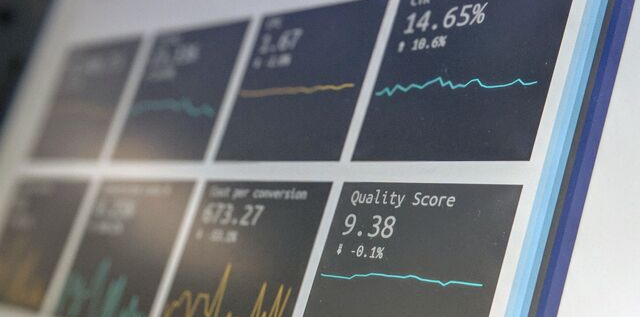# Big Data Statistical Analysis and Approximate ModelsStatistical Analysis consists often of two parts. With a real big data an analyst will start with Explorative Data Analysis, e.g.,

• Histograms

• Distribution Analysis

• Q-Q Plots (Quantile-Quantile)

• Goodness-of-Fit Tests

• Scatter Plots

• Outliers Identification and Analysis

• Diagnostics

• Transformations

• Tests of Independence

• Correlation Analysis

Based on the insight gained from the Explorative Data Analysis, the data analyst will conduct a formal statistical inference which examines a family of possible models and reach a conclusion about which model “truly” describes the data. The formal inference, particularly the Bayesians, is mostly likelihood based.

Statistical models should be considered consistently as approximations and not as true. A model is an adequate approximation to a data set if “typical” data generated under the model “look like” the real data.  The words “look like” can be measured through the selected features of the data and must be presence in the “typical” sets generated under the model.

Real big data sets can have multiple approximate models.  In the analysis of real data where theoretical assumptions are hard or even impossible to verify, it is often desired to find an adequate approximate model. For details and rigorous discussion I recommend the book “Data Analysis and Approximate Models” by Laurie Davies.